GeeksforGeeks App
Open AppBrowser
Continue

# Extract specific column from a DataFrame using column name in R

In this article, we are going to see how to extract a specific column from a dataframe using the column name in R Programming Language.

In the data.frame() we have to pass dataframe_name followed by \$ symbol followed by column name. The reason to pass dataframe_name\$ column name to data.frame() is, after extracting the data from column we have to show the data in the rows and column format. So we pass dataframe_name \$ column name to the data.frame().

Syntax of \$ operator

data.frame ( dataframe_name \$ column_name )

Example 1: In this example, we just created a data frame using data.frame() function and passed 3 vectors which holds some values. In the second step, we used \$ operator along with the data frame name inside the data.frame(). The reason behind passing dataframe_name \$ column name into data.frame() is to show the extracted column in data frame format.

## R

 `# creating a data frame with number ,``# string and binary as column names .``df1=``data.frame``(number = ``c``(1:3),``               ``string = ``c``(``"One"` `, ``"Two"` `, ``"Three"``) , ``               ``Binary = ``c``(001,010,011))`` ` `# passing column name of df1 using ``# \$ symbol to data.frame() function.``data.frame``(df1\$number) `

Output: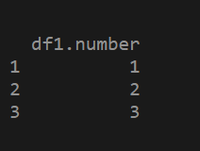Example 2: In this example, we have created 2 vectors named ranking and name with some data inside. Passed the 2 vectors into the data.frame() function as parameters and assigned it to a variable called df1, finally using \$ operator we are extracting the name column and passing it to data.frame() function for showing in dataframe format.

## R

 `# creating a vector with some values``ranking = ``c``(1 : 3) `` ` `# creating another vector with some ``# values``name = ``c``(``"Mani sharma"` `,``         ``"Devi sri prasad"` `,``         ``"Thaman SS"``) `` ` `# passing the vectors to data.frame() ``# as parameters .``df1 = ``data.frame``(ranking,name) `` ` `# Extracting name column from df1 using ``# \$ symbol``data.frame``(df1\$name) `

Output: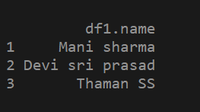Example 3: In this example, we have created 2 vectors named ranking and name with some data inside. Passing the 2 vectors into the data.frame() function as parameters. Assigned the data.frame() function into a variable named df1. Using \$ operator along with dataframe_name to extract column name and passed it into data.frame() function to show the extracted column name in data frame format.

## R

 `# creating a vector with some values``ranking = ``c``(1 : 3) `` ` `# creating another vector with some data``name = ``c``(``"Trivikram"` `, ``"RajaMouli SS"` `, ``"Puri Jagannadh"``) `` ` `# passing the vectors to the data.frame()``# function``df1 = ``data.frame``(ranking,name) `` ` `# Extracting name column from df1``# using \$ symbol and passing it to data.frame()``# as parameter .``data.frame``(df1\$name) `

Output: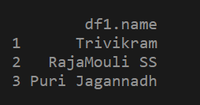## Extracting Multiple columns from dataframe

Multiple column extraction can be done through indexing.

Syntax : variable_name = dataframe_name [ row(s) , column(s) ]

Example 1: a=df[ c(1,2) , c(1,2) ]

Explanation : if we want to extract multiple rows and columns we can use c() with row names and column names as parameters. Here in the above example we have extracted 1,2 rows and 1,2 columns data from a data frame and stored into a variable.

Example 2 : b=df [ c(1,2) , c(“id”,”name”) ]

Explanation : If we want to specify column names we can give column names as parameters in c() function . In the above example we have extracted 1,2 rows of ID and name columns.

Example 1: First, we are creating a data frame with some data. Using indexing we are extracting multiple columns. In the above example, we have extracted all rows and 2 columns named number and string from df1 and storing into another variable. Finally, printing the df2.

## R

 `# creating a data frame with number``# string and binary as column names .``df1=``data.frame``(number=``c``(1:3),``               ``string=``c``(``"One"` `, ``"Two"` `, ``"Three"``) ,``               ``Binary=``c``(001,010,011))`` ` `# extracting 1 to 3 rows of string ``# and binary columns from df1``df2 = df1[``c``(1:3),``c``(``"string"``,``"Binary"``)]`` ` `# And storing the extracted data into df2``print``(df2) ``# printing the df2`

Output: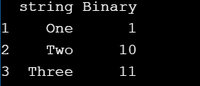Example 2: First, we are creating a data frame with some data. Using indexing we are extracting multiple columns. In the above example, we have extracted 1,2 rows and 2 columns named ranking and name from df1 and storing them into another variable. Finally, printing the df2.

## R

 `# creating a vector with some values``ranking = ``c``(1 : 3) `` ` `# creating another vector with``# some data``name = ``c``(``"Trivikram"` `, ``"RajaMouli SS"` `, ``"Puri Jagannadh"``) ``no_of_movies = ``c``(15, 10, 12)`` ` `# passing the vectors to the data.frame()``# function``df1 = ``data.frame``(ranking,name,no_of_movies) `` ` `# extracting 1,2 rows of ranking and name``# columns from df1``df2 = df1[1:2 , ``c``(``"ranking"``,``"name"``)] `` ` `# And storing the data into a variable df2``print``(df2)`

Output: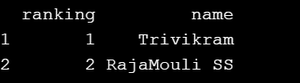Example 3: First we are creating a data frame with some data. Using indexing we are extracting multiple columns. In the above example, we have extracted all rows and 2 columns named name and no_of_movies from df1 and storing into another variable. Finally, printing the df2.

## R

 `# creating a vector with some values``ranking = ``c``(1 : 3)`` ` `# creating another vector with some values``name = ``c``(``"Mani sharma"` `, ``"Devi sri prasad"` `, ``"Thaman SS"``) ``no_of_movies=``c``(20, 30, 40)`` ` `# passing the vectors to data.frame()``# as parameters .``df1 = ``data.frame``(ranking,name,no_of_movies)`` ` `# Extracting all rows of name and ``# no_of_movies columns from df1``df2 = df1[, ``c``(``"name"``, ``"no_of_movies"``)] `` ` `# And storing into a variable called df2``print``(df2) `

Output: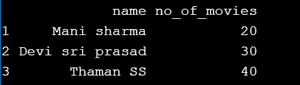My Personal Notes arrow_drop_up